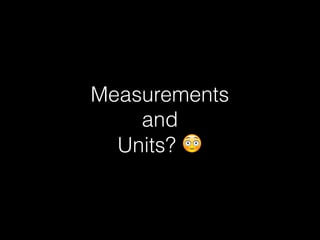Successfully reported this slideshow.

# Measurements and Units×

# Measurements and Units

This is all about "Measurement and Units", newly introduced in iOS 10 and added to the Foundation framework. WWDC2016.
You can learn how to use Measurements and create your custom units and dimensions with sample code.

This is all about "Measurement and Units", newly introduced in iOS 10 and added to the Foundation framework. WWDC2016.
You can learn how to use Measurements and create your custom units and dimensions with sample code.

### Measurements and Units

1. 1. Measurements & Units WWDC16. Meetup@Wantedly 2016/06/30
2. 2. About me Ken Tominaga The University of Tokyo Rekimoto Lab Swift Lover 😍
3. 3. Measurements  and  Units? 😳
4. 4. Nike+ Running
5. 5. Measurements and Units Added to the Foundation framework Newly introduced in iOS 10 Support for specifying Measurements and Units
6. 6. Measurement public struct Measurement<UnitType : Unit> : Comparable, Equatable { public let unit: UnitType public var value: Double public init(value: Double, unit: UnitType) }
7. 7. Calculations With Measurements let distanceTraveled = Measurement(value: 20, unit: UnitLength.kilometers) // 20.0 km let distanceToGo = Measurement(value: 1.975, unit: UnitLength.kilometers) // 1.975 km let totalDistance = distanceTraveled + distanceToGo // 21.975 km let tripleDistance = 3 * distanceToGo // 5.925 km let halfDistance = distanceToGo / 2 // 0.9875 km
8. 8. Properties of a Unit Symbol “m" Dimension “meter is a unit of length" Equivalence 1 m = 3.28084 ft
9. 9. Unit public class Unit : NSObject, NSCopying { public var symbol: String { get } public init(symbol: String) }
10. 10. Dimension Categories of units Expressed with different units • Length: km, m, ft, mi, etc. Always has a base unit let meter = UnitLength.baseUnit() Can perform conversions • km ft, m mi
11. 11. Dimension public class Dimension : Unit { @NSCopying public var converter: UnitConverter { get } public init(symbol: String, converter: UnitConverter) public class func baseUnit() -> Self }
12. 12. Dimension Instances as units Singletons for most common units International System of Units public class UnitLength : Dimension { /* Base unit - meters */ @NSCopying public class var kilometers: UnitLength { get } @NSCopying public class var meters: UnitLength { get } @NSCopying public class var feet: UnitLength { get } @NSCopying public class var yards: UnitLength { get } @NSCopying public class var miles: UnitLength { get } ... }
13. 13. Dimension
14. 14. Implicit Conversion let distanceTraveled = Measurement(value: 2, unit: UnitLength.kilometers) // 2.0 km //let distanceToGo = Measurement(value: 1.975, unit: UnitLength.kilometers) //let totalDistance = distanceTraveled + distanceToGo let distanceToGo = Measurement(value: 11.3, unit: UnitLength.feet) // 11.3 ft let totalDistance = distanceTraveled + distanceToGo // 2003.44424 m
15. 15. Next Step
16. 16. Custom Unit let syaku = UnitLength(symbol: " ", converter: UnitConverterLinear(coefficient: 10/33)) // 1 10/30 m
17. 17. Conversion baseUnit unit UnitConverter • baseUnitValue(fromValue value:) • value(fromBaseUnitValue baseUnitValue:) UnitConverterLinear • baseUnitValue = coefﬁcient * value + constant • value = (baseUnitValue - constant)/coefﬁcient
18. 18. Custom Unit let syaku = UnitLength(symbol: " ", converter: UnitConverterLinear(coefficient: 10/33)) // 1 10/33 m let lengthInShayku = Measurement(value: 10, unit: syaku) // 10.0 let lengthInYards = lengthInShayku.converted(to: UnitLength.yards) // 3.31397969193245 yd let lengthInFeet = Measurement(value: 5.3, unit: UnitLength.feet) // 5.3 ft lengthInShayku + lengthInFeet // 4.64574303030303 m baseUnitValue = 10/ 33 * = baseUnitValue / (10/33)
19. 19. Custom Dimension class UnitImpression : Dimension { static let good = UnitImpression(symbol: "👍 ", converter: UnitConverterLinear(coefficient: 1)) static let great = UnitImpression(symbol: "👏 ", converter: UnitConverterLinear(coefficient: 10)) static let wonderful = UnitImpression(symbol: "😘 ", converter: UnitConverterLinear(coefficient: 50)) static let lifeChanging = UnitImpression(symbol: "😍 ", converter: UnitConverterLinear(coefficient: 2000)) override class func baseUnit() -> UnitImpression { return good } }
20. 20. // Example - Use of Custom Dimension struct Presentation { let speaker : String let title : String let movement : Measurement<UnitLength> let duration : Measurement<UnitDuration> let impression : Measurement<UnitImpression> var movementRate: Measurement<UnitSpeed> { let distanceInMeters = movement.converted(to: .meters) let durationInSeconds = duration.converted(to: .seconds) return Measurement( value: distanceInMeters.value / durationInSeconds.value, unit: UnitSpeed.metersPerSecond) } }
21. 21. // Example - Use of Custom Dimension let presentation = Presentation( speaker: "ken0nek", title: "Measurements & Units", movement: Measurement(value: 60, unit: UnitLength.centimeters), duration: Measurement(value: 10, unit: UnitDuration.minutes), impression: Measurement(value: 100, unit: UnitImpression.lifeChanging)) presentation.movementRate // 0.001 m/s presentation.impression // 100.0 😍 presentation.impression.converted(to: UnitImpression.baseUnit()) // 200000.0 👍
22. 22. Formatting Is Hard Country Expected String Japan “5 km" United States “3.1 mi” Egypt “‫كم‬ ٥” China “5 "
23. 23. MeasurementFormatter New formatter Measurements and Units Locale-aware formatting
24. 24. MeasurementFormatter public class MeasurementFormatter : Formatter { public var unitOptions: MeasurementFormatter.UnitOptions public var unitStyle: Formatter.UnitStyle @NSCopying public var locale: Locale! @NSCopying public var numberFormatter: NumberFormatter! public func string(from measurement: Measurement<Unit>) -> String public func string(from unit: Unit) -> String }
25. 25. Unit Options Formats preferred unit of locale by default Takes purpose into account
26. 26. Unit Options UnitOptions Measurement Locale Example String .providedUnit value: 5, unit: .kilometers “en_US” “5 km" .naturalScale value: 1000, unit: .meters “fr_FR” “1 km" .temperatureWithoutUnit value: 90, unit: .fahrenheit “en_US” “90°"
27. 27. MeasurementFormatter // Current Locale -> ja_JP // This code will not work in Xcode8 beta currently :( let formatter = MeasurementFormatter() let distance = Measurement(value: 5, unit: UnitLength.miles) formatter.string(from: distance) // 8.04672 km let syaku = UnitLength(symbol: " ", converter: UnitConverterLinear(coefficient: 10/33)) let syakuDistance = Measurement(value: 660, unit: syaku) formatter.string(from: syakuDistance) // 0.2 km
28. 28. Wrap Up Support for handling Measurements and Units Don’t have to care about localizing and formatting Measurement Unit Dimension MeasurementFormatter UnitConverter
29. 29. Thank you
30. 30. References Measurements and Units - WWDC 2016 - Videos - Apple Developer Measurement - Apple Developer Documentation Unit - Apple Developer Documentation Dimension - Apple Developer Documentation UnitConverterLinear - Apple Developer Documentation MeasurementFormatter - Apple Developer Documentation About Internationalization and Localization
31. 31. Bug Reports The string() method of the MeasurementFormatter... | Apple Developer Forums Swift Compiler on iOS 10 does not properly ﬁnd MeasurementFormatter.string(from:) method